Type of triangle formed

Chapter 7 Class 10 Coordinate Geometry
Concept wise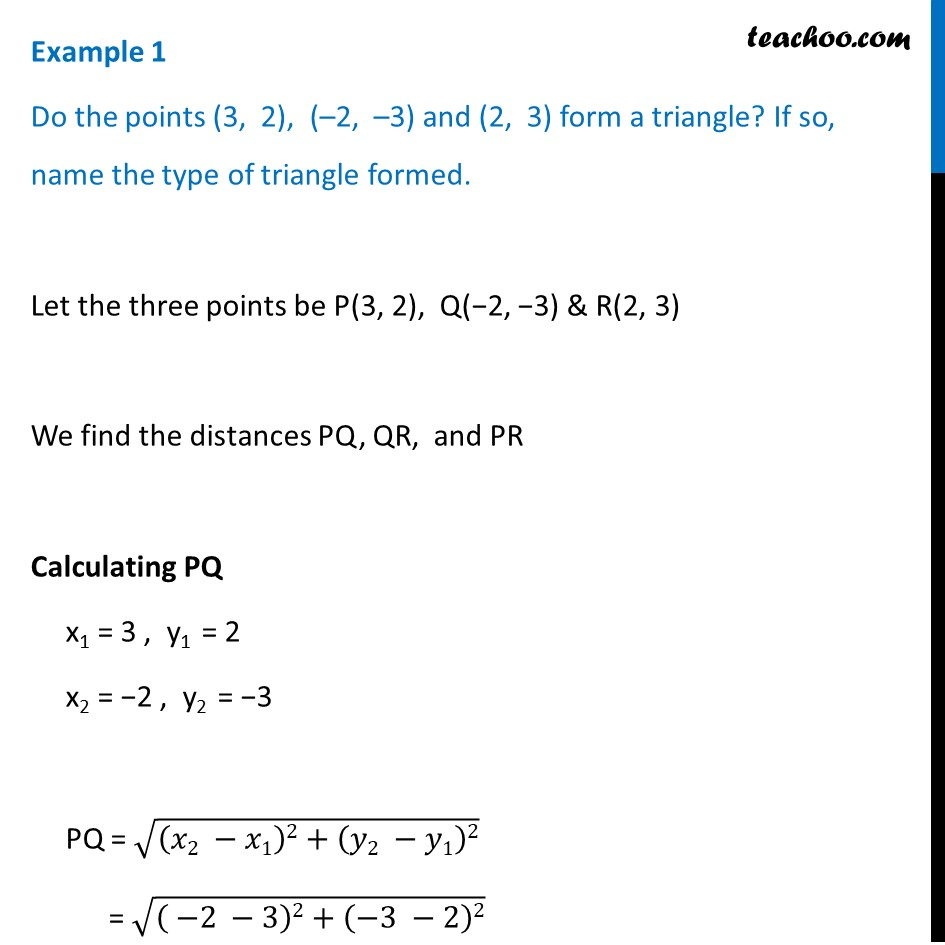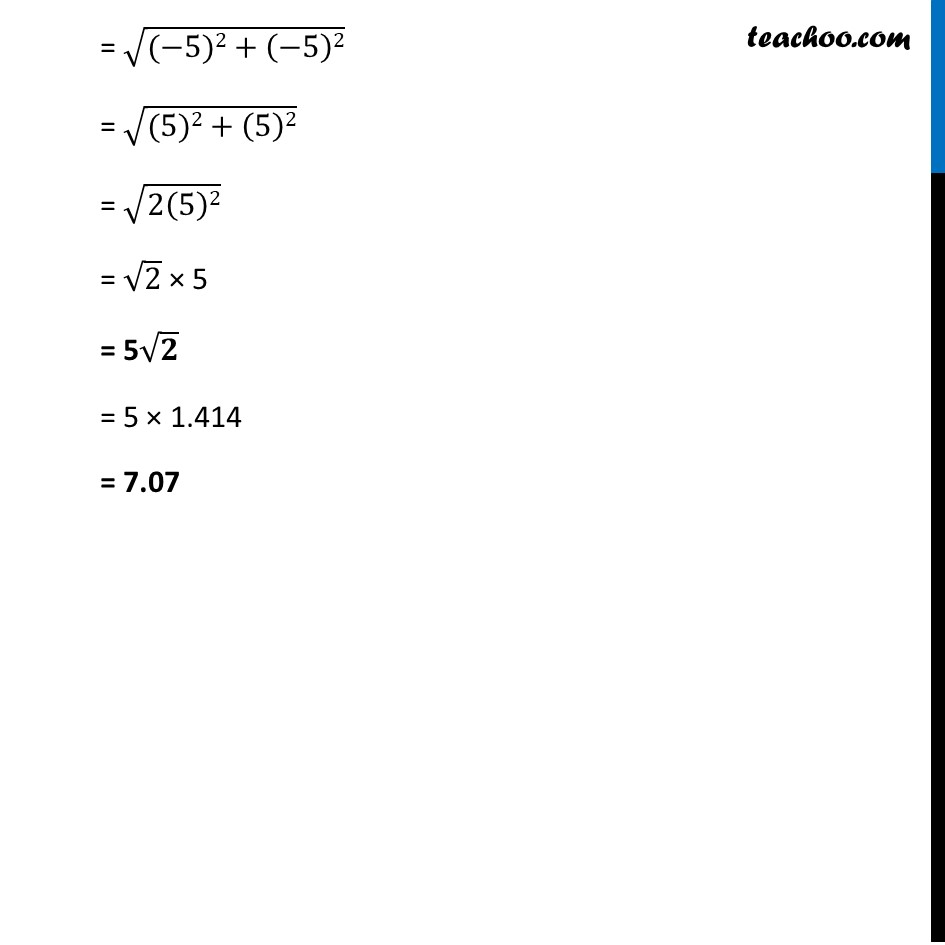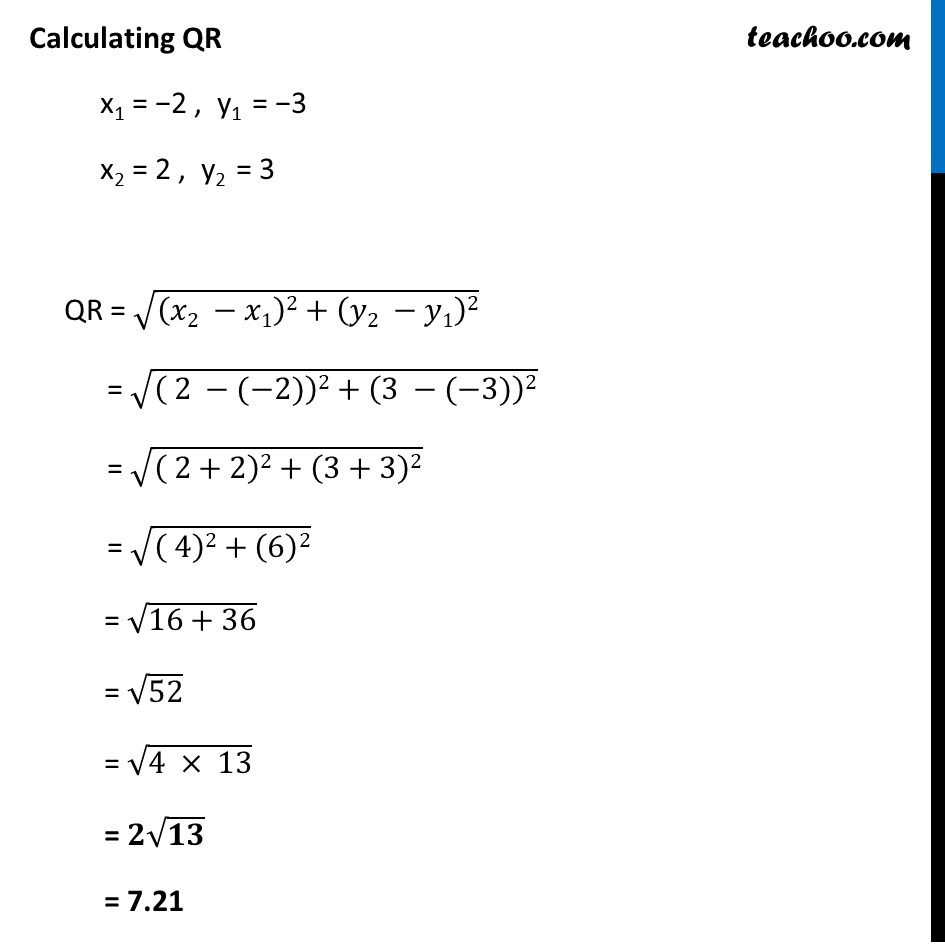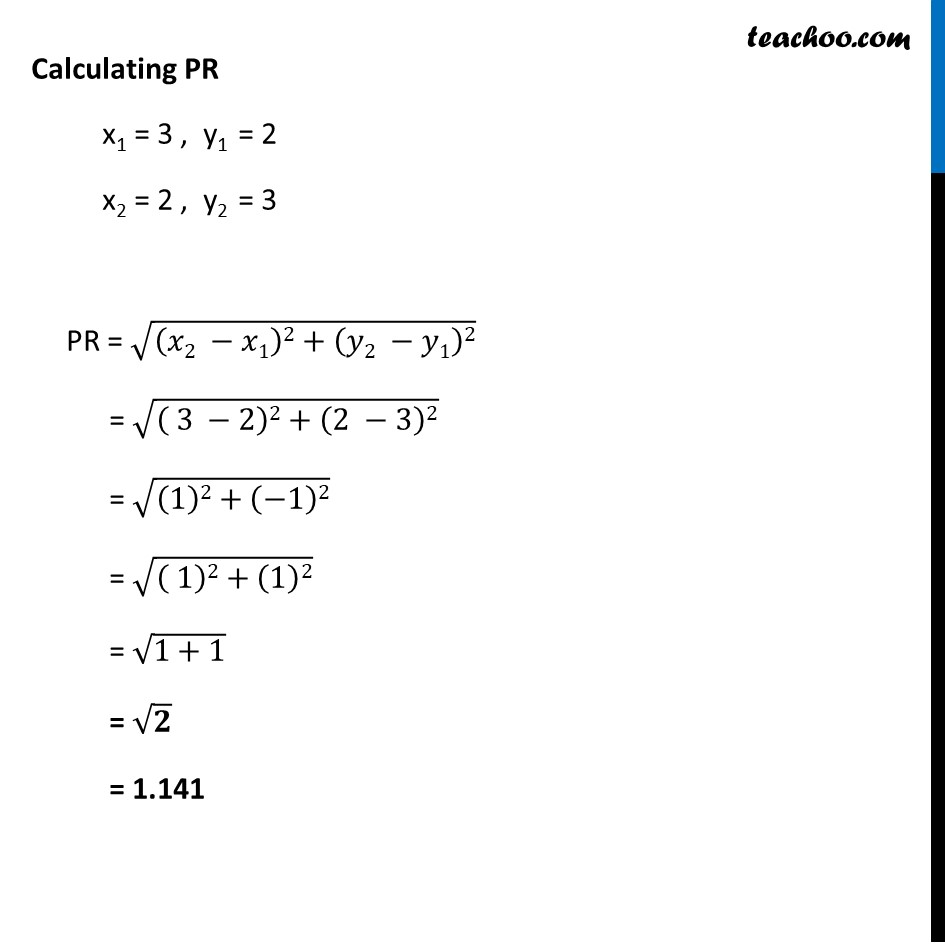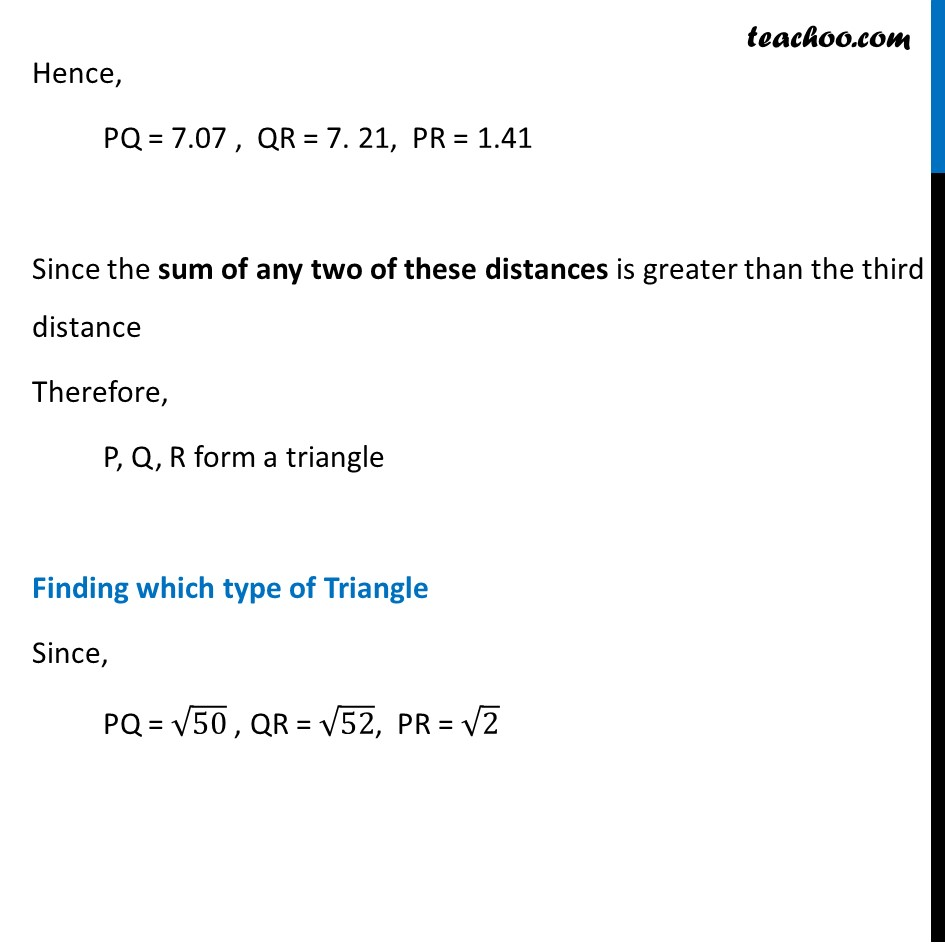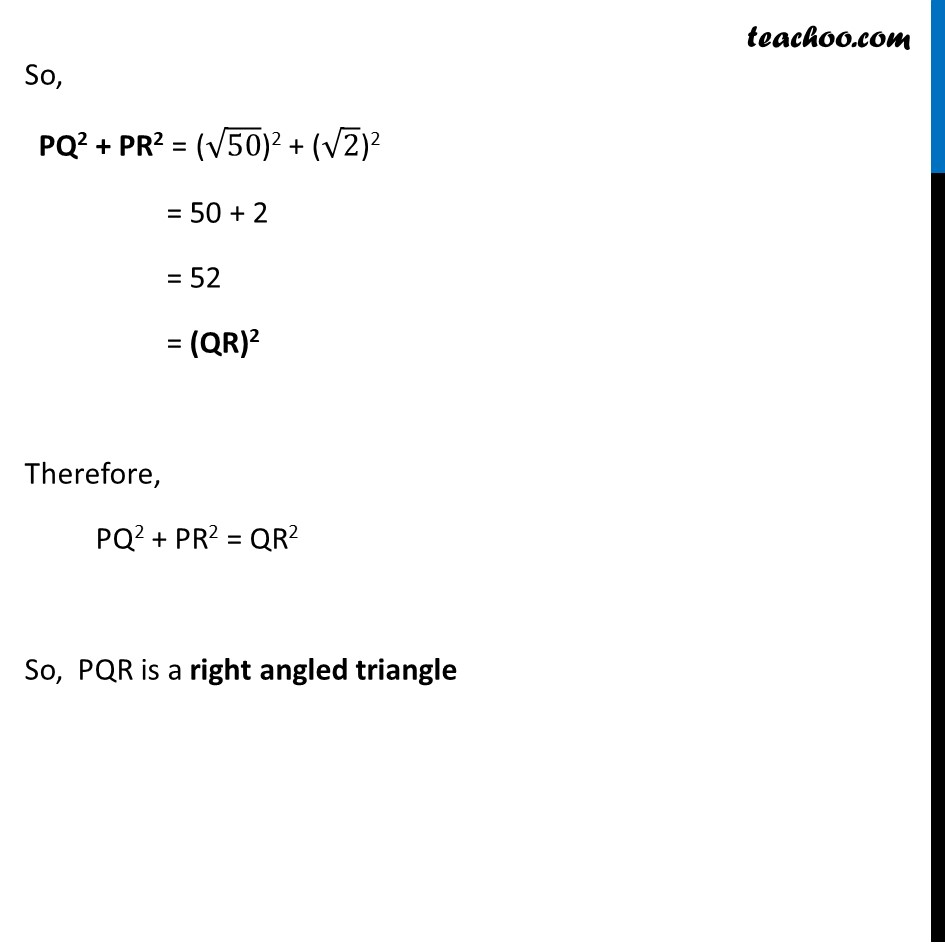Introducing your new favourite teacher - Teachoo Black, at only ₹83 per month

### Transcript

Example 1 Do the points (3, 2), (–2, –3) and (2, 3) form a triangle? If so, name the type of triangle formed. Let the three points be P(3, 2), Q(−2, −3) & R(2, 3) We find the distances PQ, QR, and PR Calculating PQ x1 = 3 , y1 = 2 x2 = −2 , y2 = −3 PQ = √((𝑥2 −𝑥1)2+(𝑦2 −𝑦1)2) = √(( −2 −3)2+(−3 −2)2) = √((−5)2+(−5)2) = √((5)2+(5)2) = √(2(5)2) = √2 × 5 = 5√𝟐 = 5 × 1.414 = 7.07 Calculating QR x1 = −2 , y1 = −3 x2 = 2 , y2 = 3 QR = √((𝑥2 −𝑥1)2+(𝑦2 −𝑦1)2) = √(( 2 −(−2))2+(3 −(−3))2) = √(( 2+2)2+(3+3)2) = √(( 4)2+(6)2) = √(16+36) = √52 = √(4 × 13) = 𝟐√𝟏𝟑 = 7.21 Calculating PR x1 = 3 , y1 = 2 x2 = 2 , y2 = 3 PR = √((𝑥2 −𝑥1)2+(𝑦2 −𝑦1)2) = √(( 3 −2)2+(2 −3)2) = √((1)2+(−1)2) = √(( 1)2+(1)2) = √(1+1) = √𝟐 = 1.141 Hence, PQ = 7.07 , QR = 7. 21, PR = 1.41 Since the sum of any two of these distances is greater than the third distance Therefore, P, Q, R form a triangle Finding which type of Triangle Since, PQ = √50 , QR = √52, PR = √2 So, PQ2 + PR2 = (√50)2 + (√2)2 = 50 + 2 = 52 = (QR)2 Therefore, PQ2 + PR2 = QR2 So, PQR is a right angled triangle